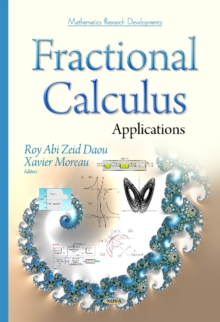Supporting your high street Find out how »
• My AccountFractional Calculus : Applications Hardback

Edited by Roy Abi Zeid Daou, Xavier Moreau

Description

After presenting the first volume of this two-volume book, presenting a lot of mathematical and theoretical studies and research related to non-integer calculus, the second volume illustrates applications related to this domain.

This volume is made up of 11 chapters. The first chapter presents the heuristic power of the non-integer differential operators in physics starting from the chaos to the emergence, the auto-organizations and the holistic rules.

The second chapter shows the dynamics of the fractional order chaotic systems along with some applications.

The third chapter represents the pressure control of gas engines by non-integer order controllers by showing a novel trend in the application of the fractional calculus to automotive systems.

Chapter 4 shows the way to model fractional order equations using state space modeling along with some applications.

Another application related to this domain is the thermal diffusive interface.

Chapter 5 shows the analysis of a semi-infinite diffuse plane medium along with the equations that model this medium, and some frequency and time domain responses.

However, Chapter 6 treats this problem by controlling this plant using the well-known CRONE controller.

Chapter 8 presents the adaptive second-order fractional sliding mode control with an application to a water tanks level system.

Chapter 9 treats the mechanical aspect by showing the features of the fractional operators applied to this domain.

Also, Chapter Nine presents the theory of diffusive stresses based on the fractional advection-diffusion equation.

The modeling of drug diffusion during general anesthesia using Fractional Calculus is shown in Chapter 10 and is considered as another application related to the biomedical field.

Finally, Chapter 11 represents an overview of the fractional fuzzy controllers by showing the analysis, the synthesis and the implementation of this module.

To sum up, this second volume presents applications of fractional calculus in several engineering domains as the thermal, the automotive, the mechanical, the biomedical and much more.

Note that this volume was preceded by a first volume that focuses on the mathematical and theoretical aspects of fractional calculus.

Information

• Format: Hardback
• Pages: 241 pages
• Publisher: Nova Science Publishers Inc
• Publication Date:
• Category: Calculus & mathematical analysis
• ISBN: 9781634632218

£161.99

£130.15

on all orders

Pick up orders

from local bookshops